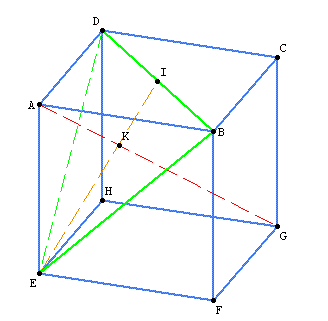Compute the volume of a cube region

Hi,

I would like to compute the volume $V$ of the lower polyhedron (that does not contain vertex A) let's call it $poly_G$. (let's call $poly_A$ the upper polyhedron that does not contain G). Size is $a=AB=BF=...$We can compute $V_{poly_A}$ by introducing K and I points and using Pythagore's theorem and median's properties . I can do that by hand using $V_{poly_G}=V_{cube}-V_{poly_A}$

I want to compute the volume with sage, I have tried this code using volume integrations but I am not sure about the result at all... I look at first for the normal vector of plane DBE (cross product) which gives the equation plane $x-y+z$. Then I compute using triple integrations.

x=var('x')
y=var('y')
z=var('z')
a=var('a')
u=vector([a,a,0])
v=vector([0,a,a])
print u.cross_product(v)
intz = integrate(1,z,0,a-y-x)
inty = integrate(intz,y,0,a-x)
intx = integrate(inty,x,0,a)
print intx

I would like to know if there are elegant ways of computing this kind of problem in sage ? And in addition, if this require few lines, how can I display the above figure ? Sorry if this looks simple, I am new to Sage.

Thanks,

edit retag close merge delete

Sort by » oldest newest most voted

You can construct the 3-dimensional cube as follows:

sage: P = polytopes.n_cube(3) ; P
A 3-dimensional polyhedron in ZZ^3 defined as the convex hull of 8 vertices
sage: P.vertices()
(A vertex at (-1, -1, -1),
A vertex at (-1, -1, 1),
A vertex at (-1, 1, -1),
A vertex at (-1, 1, 1),
A vertex at (1, -1, -1),
A vertex at (1, -1, 1),
A vertex at (1, 1, -1),
A vertex at (1, 1, 1))

Then construct the polyhedron with the last vertex removed:

sage: Q = Polyhedron(P.vertices()[:-1]) ; Q
A 3-dimensional polyhedron in ZZ^3 defined as the convex hull of 7 vertices
sage: Q.vertices()
(A vertex at (-1, -1, -1),
A vertex at (-1, -1, 1),
A vertex at (-1, 1, -1),
A vertex at (-1, 1, 1),
A vertex at (1, -1, -1),
A vertex at (1, -1, 1),
A vertex at (1, 1, -1))

Then compute its volume:

sage: Q.volume()
20/3

In our case, the length of the edge is 2. If you want to replace it by a, it suffice to multipliy the result by by (a/2)^3:

sage: a = var('a')
sage: Q.volume()* (a/2)^3
5/6*a^3

If you want to have a plot of the polyhedron Q, just do:

sage: Q.plot()
more